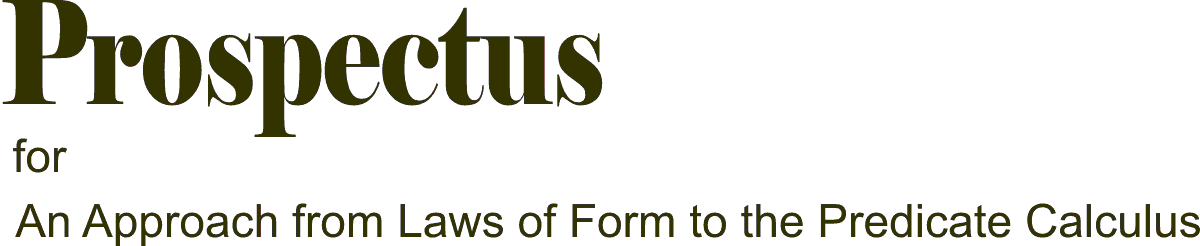As the main text of 'An approach...' is rather long and makes use of a notation which will be strange to most readers, and will take quite a bit of getting used to, I have cooked up this introductory example or 'prospectus' with the aim of enticing the unwary general reader into the main text. Some of course will be unenticed - there is nothing I can do about that. I am only interested in the person who feels that there could be something here that is worth looking into. To this person I tender my apologies that I cannot explain the whole thing better, and make it easier to assimilate. I feel sure that sooner or later others will follow and make a better job of it.

So let's jump in and see what we can do with a typical problem of predicate logic. This one is taken from the online text 'for all x: An Introduction to Formal Logic' by P.D.Magnus, page 138. (You will also find relevant material on pages 67 and 155.)

### Problem

Suppose we are given the following (the premise):

There is someone who likes everyone that likes everyone that he or she likes

Prove that we may legitimately draw the following conclusion from the premise:

There is someone who likes him or herself.

Before embarking on our quest for a proof, let us clarify the premise. It is meant that there is someone (let's call them A) who likes everyone that likes everyone that A likes. Otherwise, it is not quite clear who the he or she is that is mentioned near the end of the statement.

### Stage 1 Transcription of premise and conclusion into the notation of the conventional predicate calculus

As the premise is somewhat convoluted, we break down the transcription into several stages.

(i) The statement 'y is someone who likes everyone that x likes' may be rephrased as follows:

for all z, (x likes z) implies (y likes z)

which can be written in abbreviated formulaic form as

∀z ((xLz)→(yLz))

(ii) Building on this, the statement

if y is someone who likes everyone that x likes, then x likes y

may be transcribed as

(∀y (∀z((xLz)→(yLz)))) → xLy

(iii) Finally, the premise as a whole can be rephrased as

there exists an x, such that the statement in paragraph (ii) is true

∃x ( ∀y ( ((∀z((xLz)→(yLz))) → xLy ) ) ).

(iv) The conclusion is relatively easy to transcribe:

∃x xLx

### Stage 2 Transcription into the CLS-calculus

(This stands for 'the calculus of Circles, Letters and Subscripts'.) We transcribe our formulae using the following rules:

1. the two-place predicate 'x likes y' or xLy is written as Lxy (in general: a subscripted upper-case letter).
2. 'A and B' or A∧B is written simply as AB
3. 'not A' or ¬A is written as A enclosed in a circle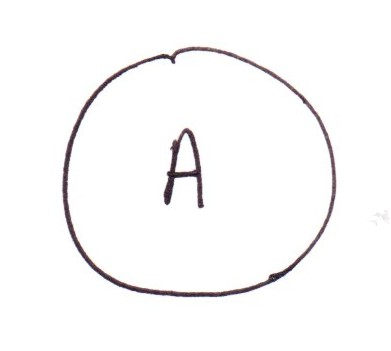4. 'A or B' or A∨B is written as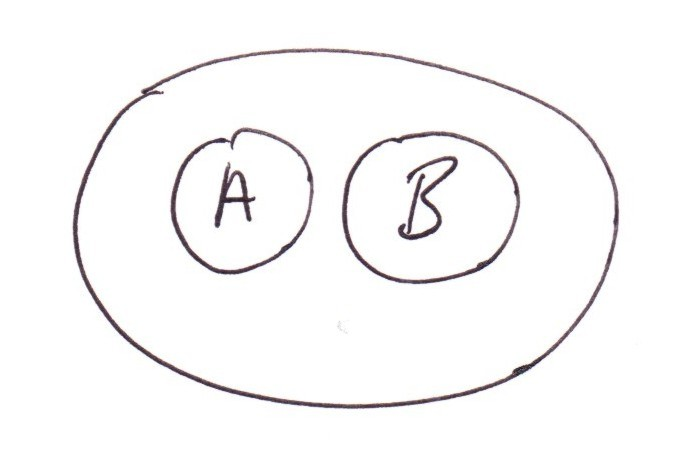5. 'if A then B' or 'A implies B' or A→B is written as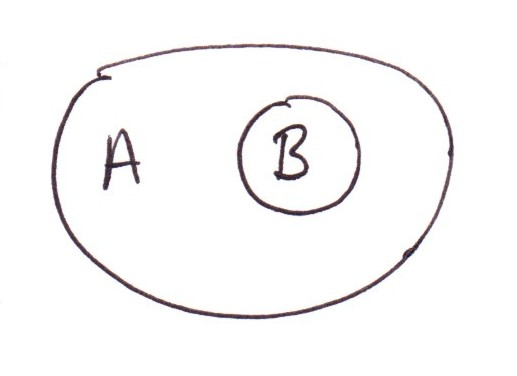By i and ii this means the same as 'not (A and not-B)'
Alternatively, by i and iv, it means the same as 'either B or not-A'

Following these prescriptions, (xLz)→(yLz) becomes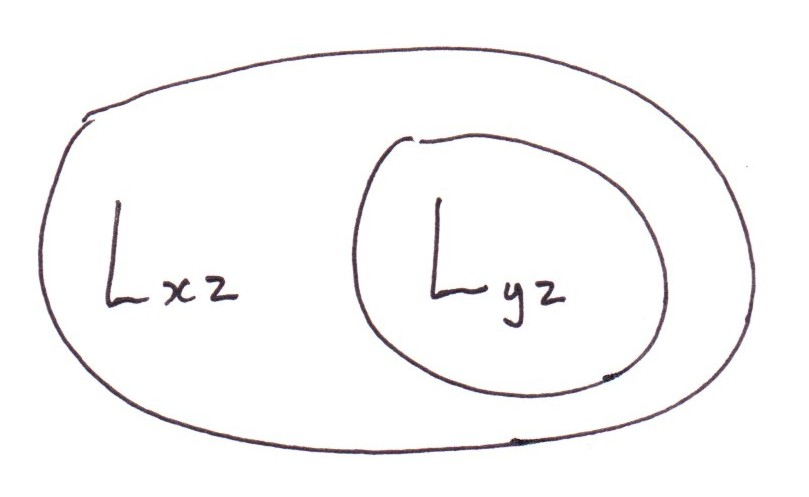We deal with the quantifier ∀z by writing it beside the expression: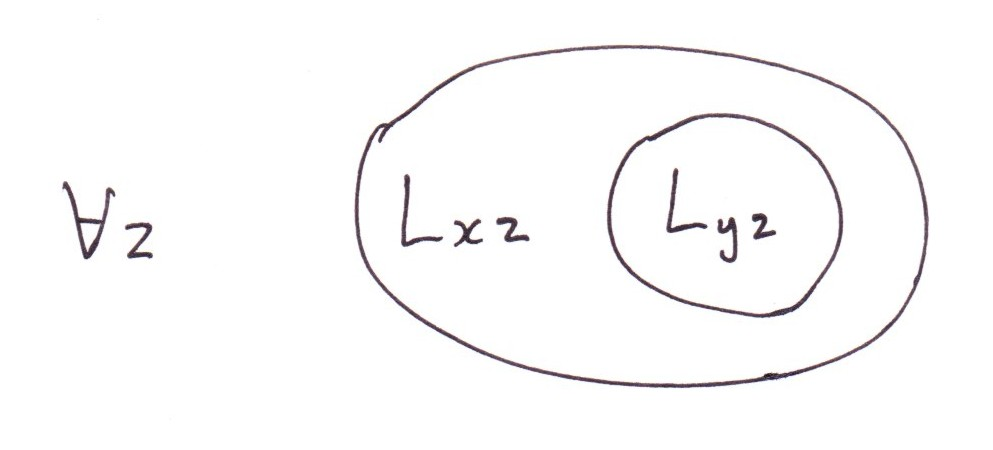(∀z((xLz)→(yLz))) → xLy becomes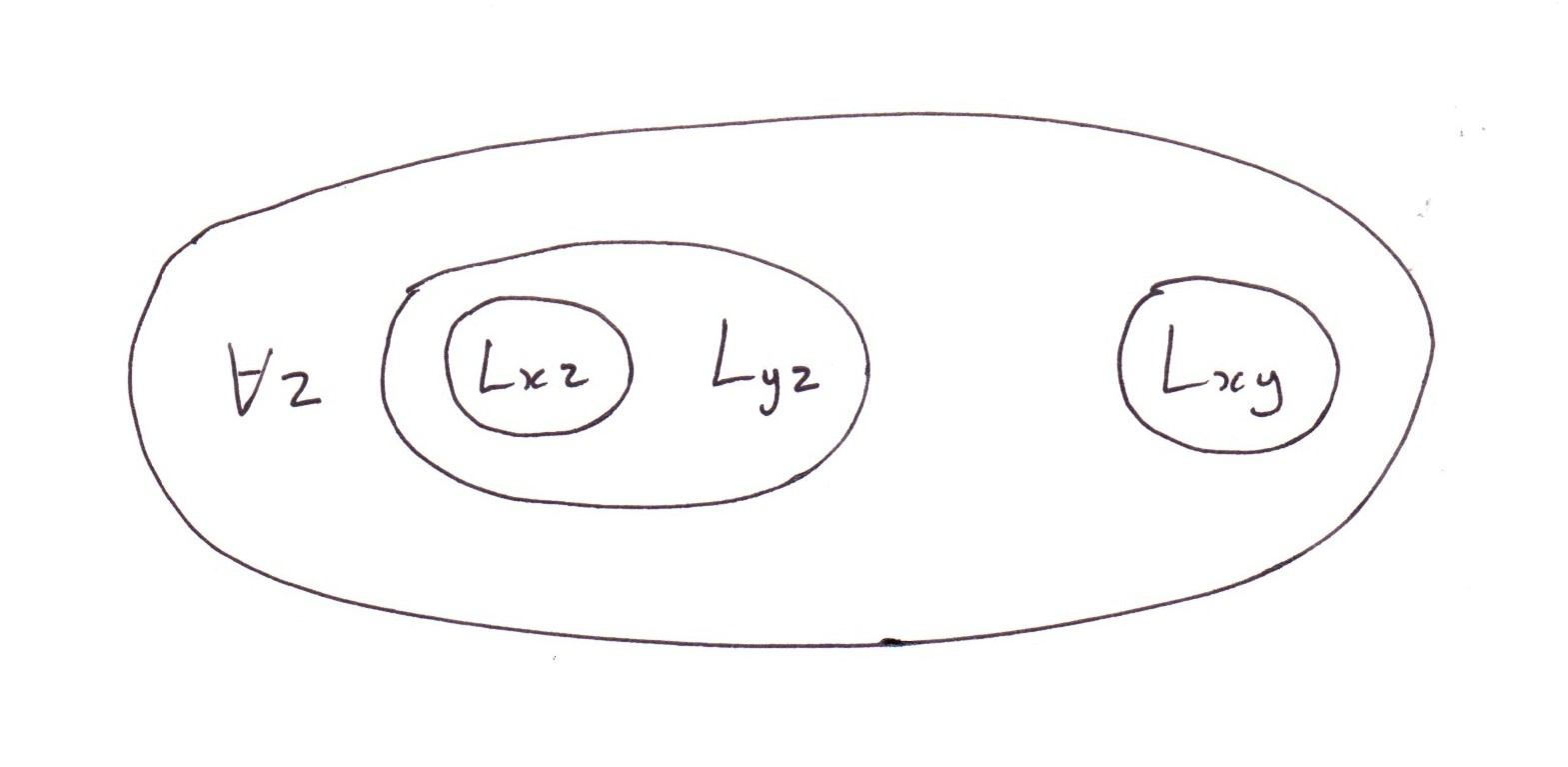Again, we deal with the quantifiers ∃ and x.∀y by writing them beside the expression: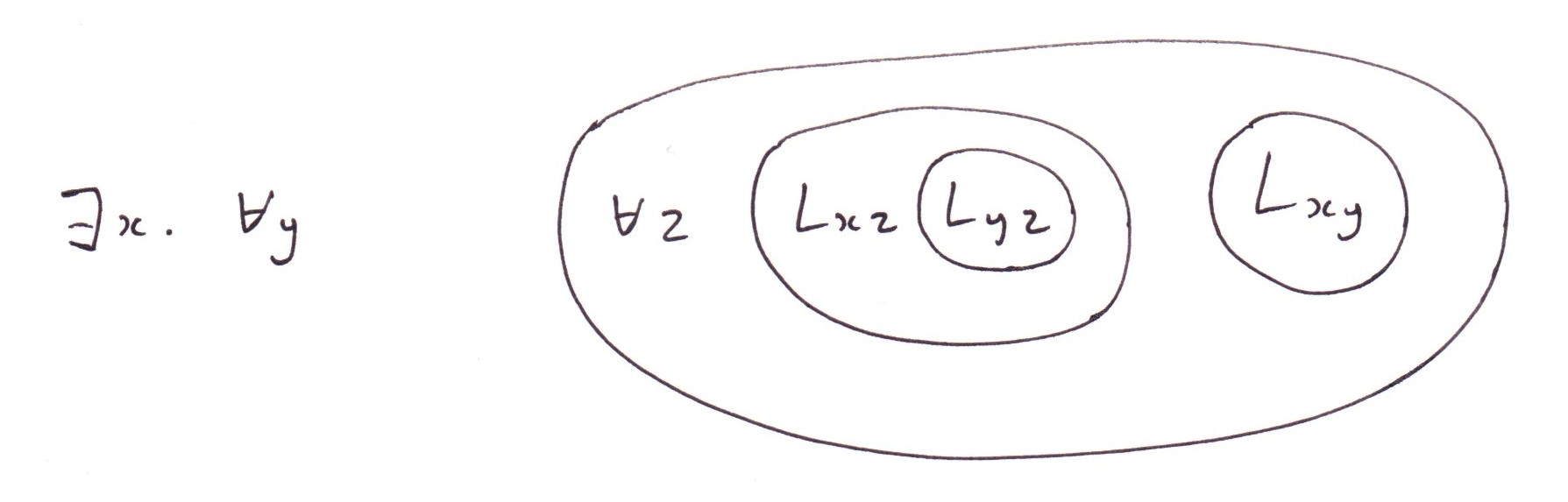We write the dot between ∃x and ∀y to indicate that the quantifier ∀y is 'inside' the ∃x; we're saying 'there exists an x such that for all y...'. If they were the other way round, like so

∀y.∃x ...

the meaning would be different; it would be saying 'for all y there is an x such that...'

### Stage 3 Get rid of the quantifiers 

We start at the outside of our expression and work inwards. The first quantifier we come across is ∃x. Our attitude is this. We're told, there is an x such that blah blah. Well let's call that x (or one of those x's, if there is more than one of them) x. Then we rewrite the expression, leaving out the ∃x and replacing each occurrence of x by x, thus: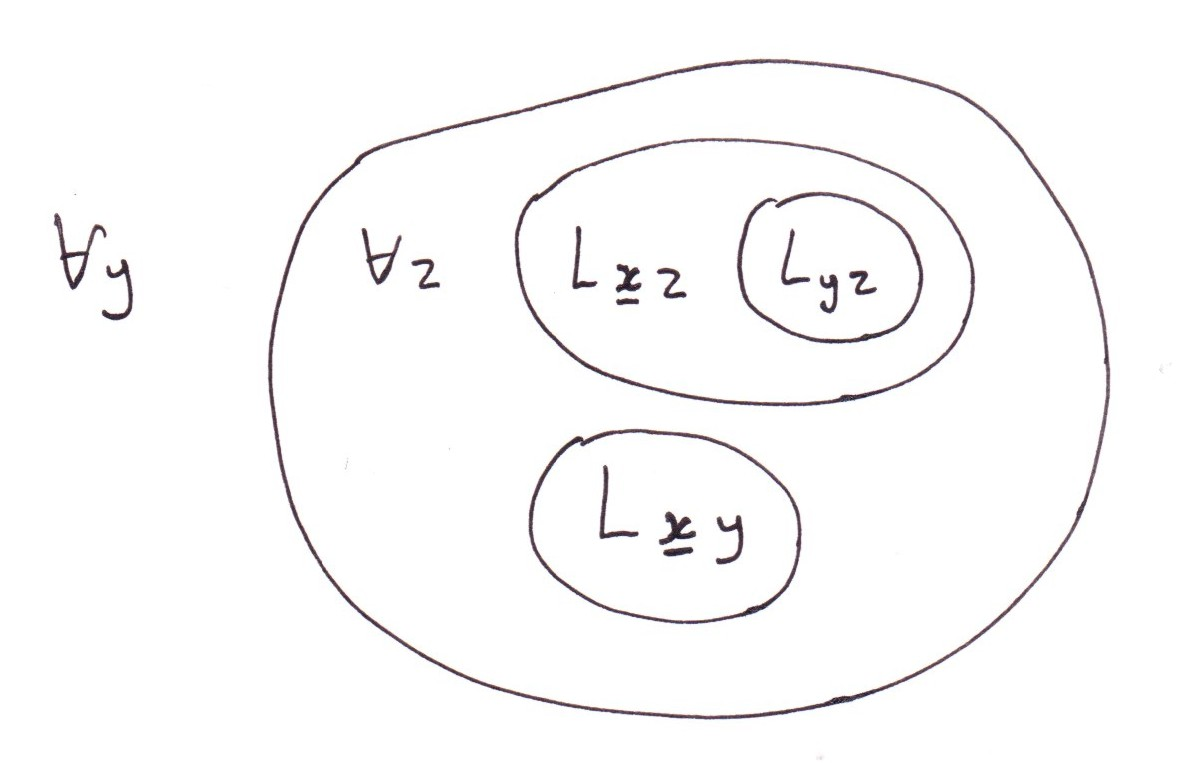The outermost remaining quantifier is ∀y. We deal with it by leaving it as it is, for the time being.

Next outermost is ∀z. However, ∀z is not on the outside of our expression. To get to the outside, it would have to cross a boundary. Well, that's OK, except there is just one little extra rule that we haven't mentioned yet. When an ∀ crosses a boundary, it becomes an ∃; and when an ∃ crosses a boundary, it becomes an ∀.

(You can see that this is perfectly reasonable, as follows. To say 'it is not the case that for all x, P is true of x' is the same as saying 'there is an x such that P is not true of x'. Similarly, to say 'it is not the case that there exists an x such that P is true of x' is the same as saying 'for all x, P is not true of x'.)

So now we have: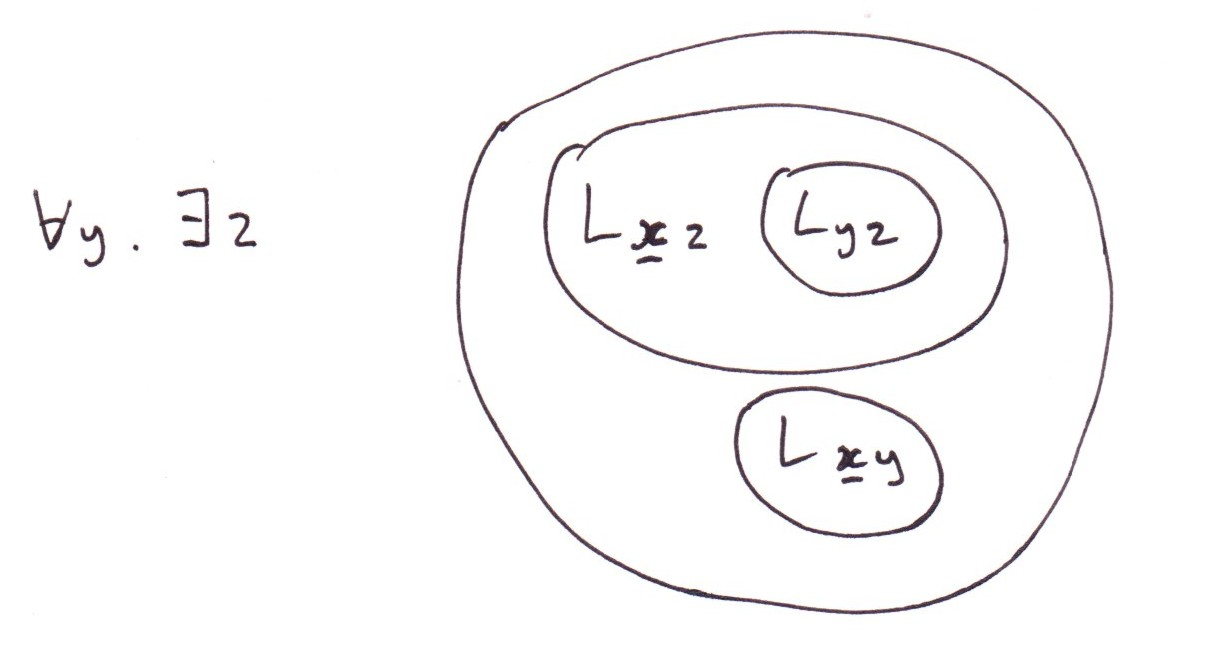OK, now the quantifier ∃z is on the outside, saying there is a z such that blah blah; so why don't we just call that z that does the trick, z ? There's just one little snag: that particular z called z is allowed to depend on y. 'For each y, there is a z such that...'   The point is, there doesn't have to be a universal z that does the trick for every possible choice of y: z can be tailored to y. We indicate this by writing z with a subscript y, thus:

zy

We then proceed to rub out ∃z, and replace each occurrence of z in the expression by a subscripted, bold z: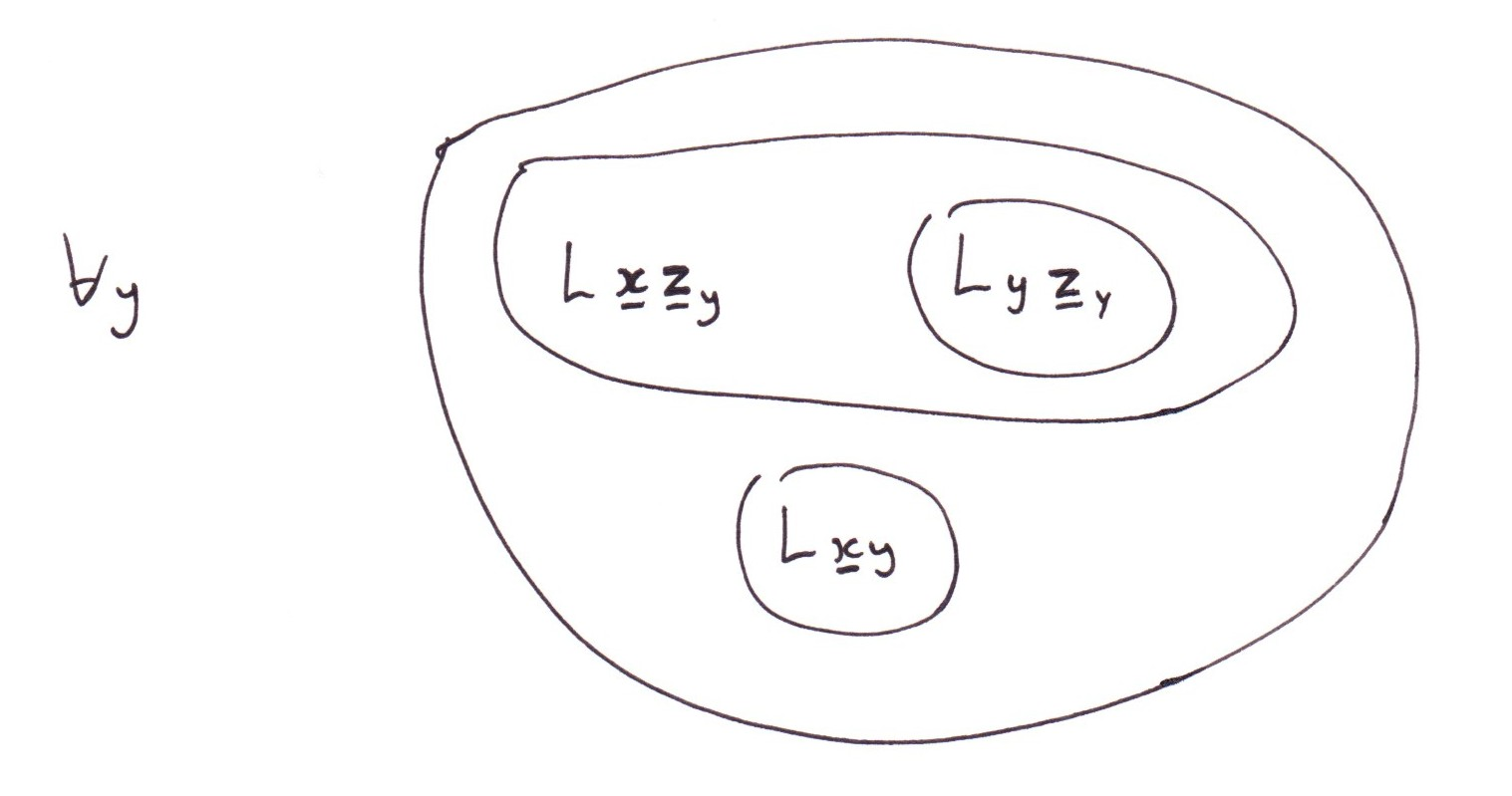Finally, we erase the ∀y. Any lower-case letter that has not been emboldened by this late stage we assume to be a 'for all' type of letter. The final result of Stage 3: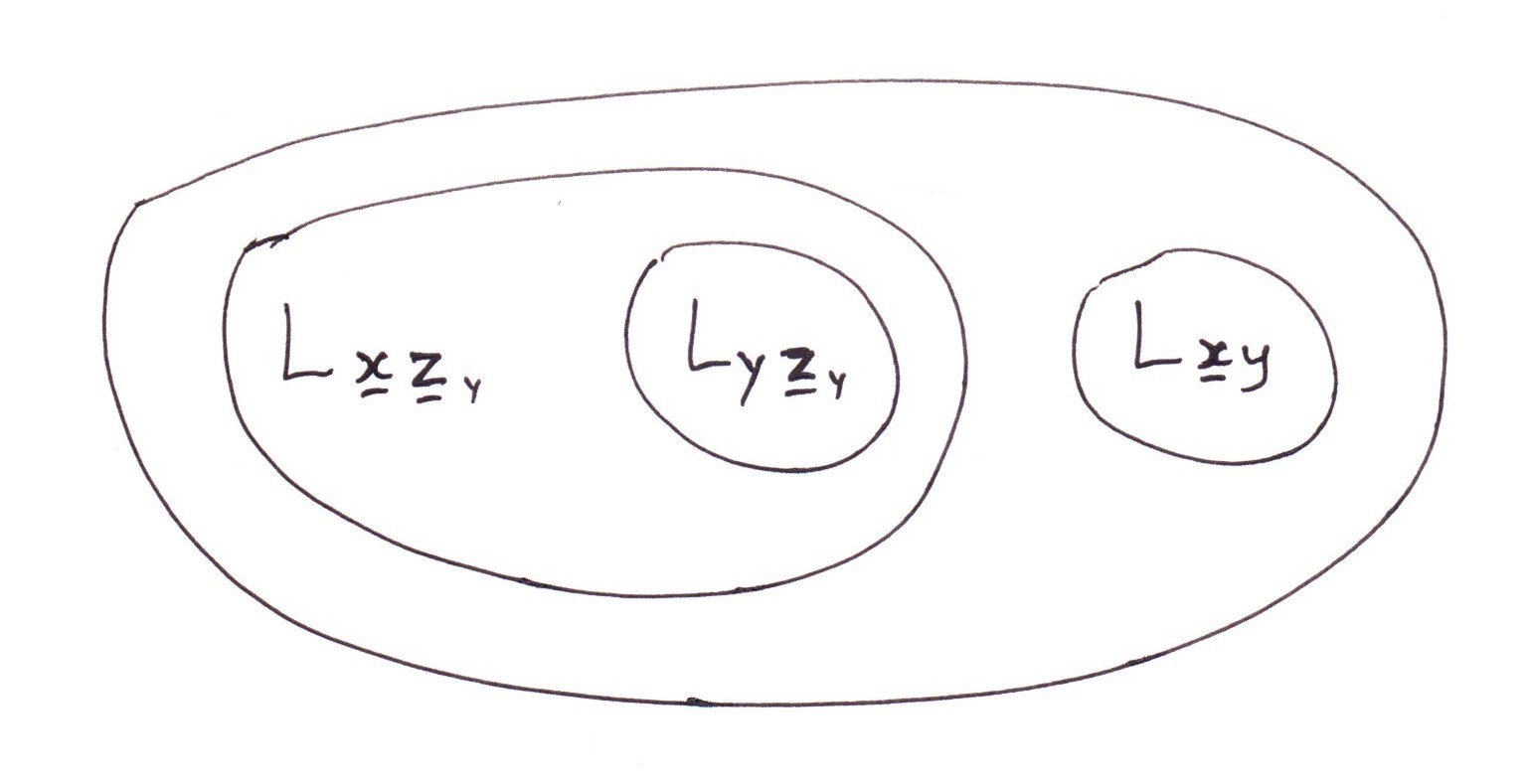### Stage 4 The Process of Deduction

The above expression transcribes our premise, 'There is someone (call them A) who likes everyone that likes everyone that A likes'. Our task now is to draw inferences from it. The challenge is to do this not by thinking but by blindly calculating. In the calculation we are allowed to transform the premise-expression according to certain rules. (Think of these as analogous to the rules of algebra e.g. you can multiply top and bottom by the same thing.)

1. You can perform any of the allowed moves of the Circle-and-Letter Calculus (CL-calculus for short), treating any subscripted upper-case letter (what we will call an 'atom' for short) just as if it were an ordinary letter in the CL-calculus. To fully understand what this means you will have to study the main part of The Markable Mark. For now, we'll just say that any legal CL-move can be built up by combining instances of the following six primitive moves, or replacements. They are grouped into three pairs:

1.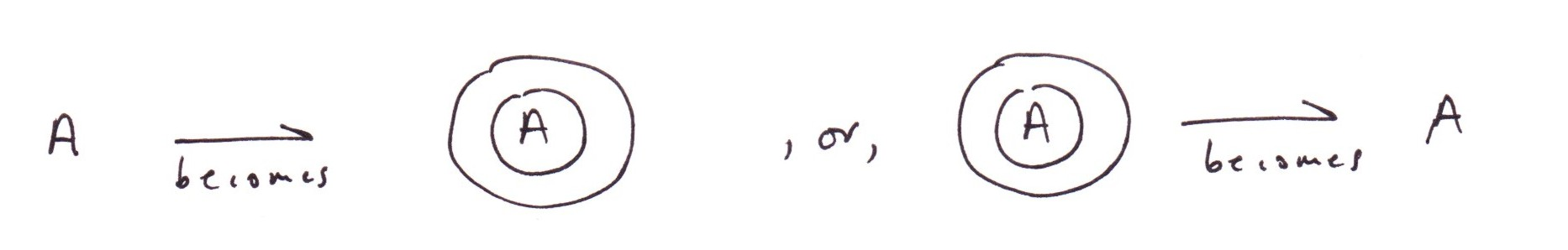These moves are called 'wrap' and 'unwrap' respectively
2.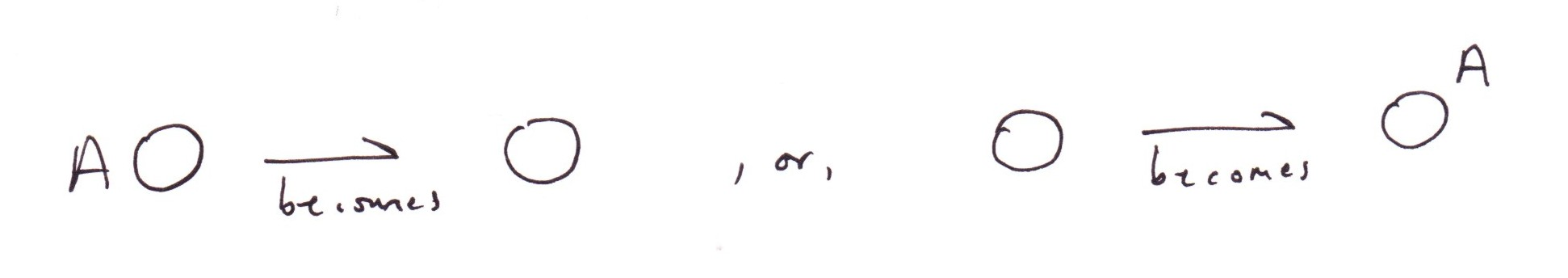These moves are called 'delete' and 'undelete' respectively
3.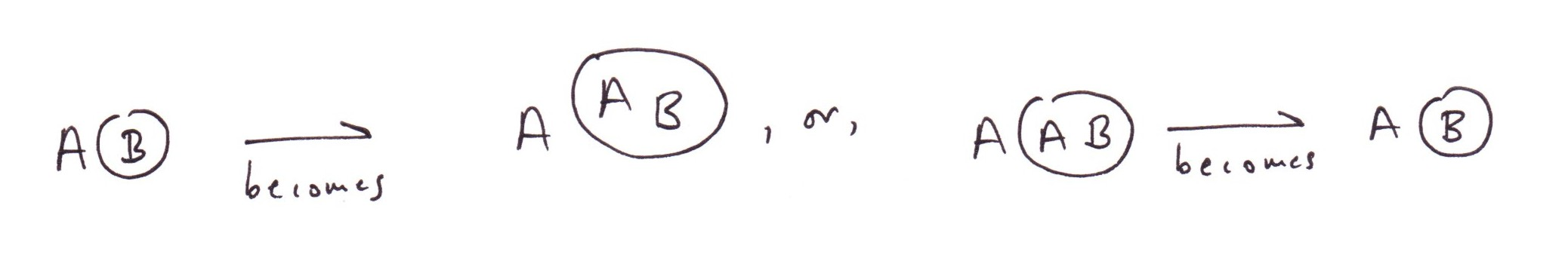These moves are called 'copy' and 'uncopy' respectively

2. You can perform a new kind of move, exclusive to the Circle-Letter-and-Subscript Calculus (CLS-calculus) called theta-copy. Given an expression, you can take any of its factors, make a copy of it in the space beside the original expression, then subject this copy to a substitution. Neglecting certain subtleties (which are covered in the main text of 'An approach...'), a substitution fixes its attention on an unbold or still variable lower-case letter, which we will call the replacee; selects a replacement letter (which can be either another variable letter, or a bold letter, or a subscripted bold letter); then goes through the factor replacing each occurrence of the replacee by the replacement. The result of doing two substitutions in succession is also regarded as a substitution.

So let's have another look at our expression, but now bearing these possible moves in mind:If we restrict attention to CL-moves there is nothing useful to be done because no two 'atoms' (i.e. subscripted upper-case letters) are the same. We need to get to work on the expression with theta-copy. We note that there are no factors other than the whole expression, so the whole expression needs to be copied, then the copy subjected to a substitution. y is the only letter that is still variable (the others are 'fixed' i.e. bold) so we select y as replacee. As replacement we could choose anything; but as our aim is to cause some atoms to become identical, the obvious choice is x. Replacing, in the copy, each occurrence of y by x, we obtain: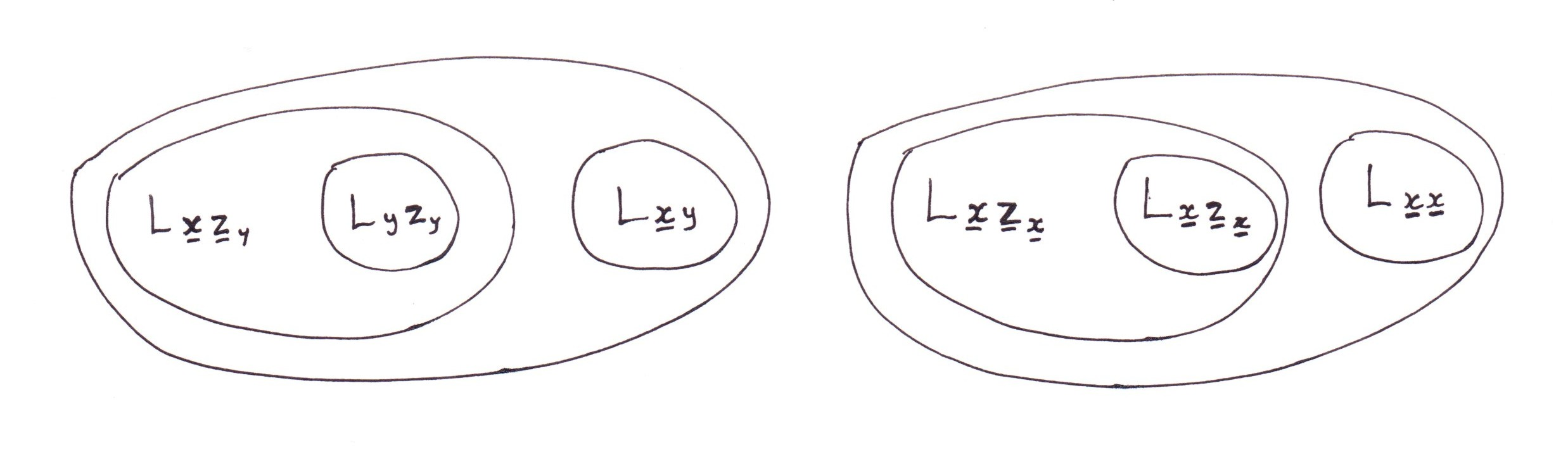Two of the atoms in the second factor have indeed become identical. This second factor now has the form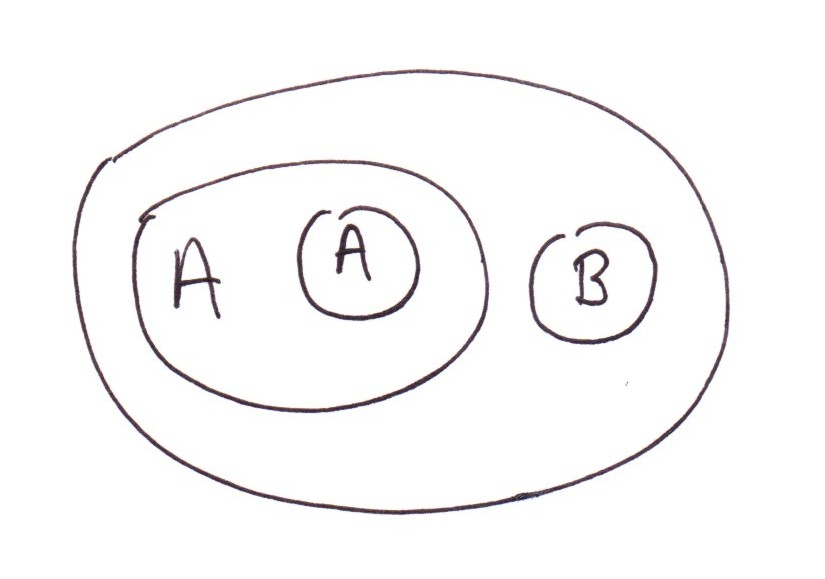(where A stands for Lxzx, B for Lxx). Now, it is a theorem of the CL-calculus that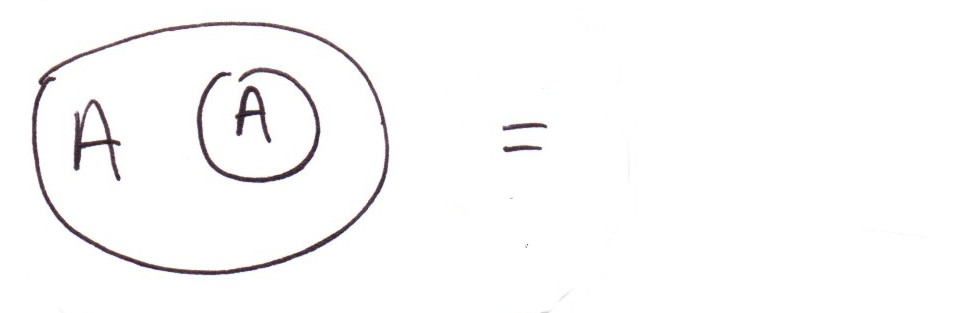in other words, any expression having the form of the expression on the left is equivalent to the void expression. (The theorem may be proved by performing the moves uncopy, delete, unwrap in succession.)

So, substituting the void,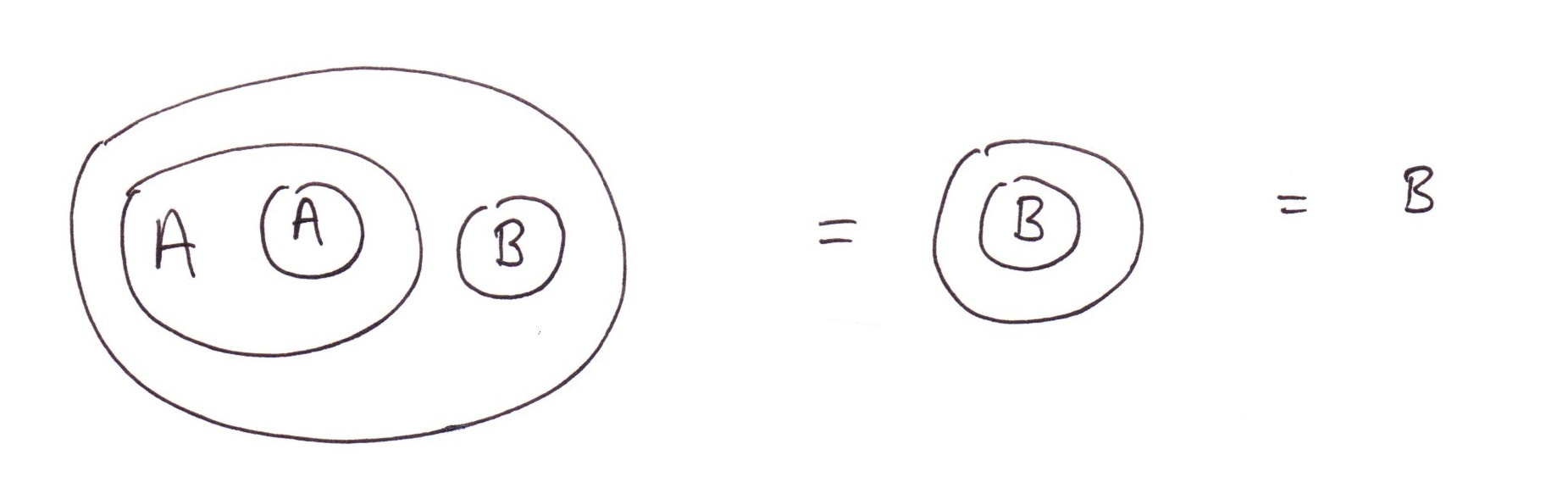where the second equivalence is an application of 'unwrap'. Thus our whole initial expression (the premise) is equivalent to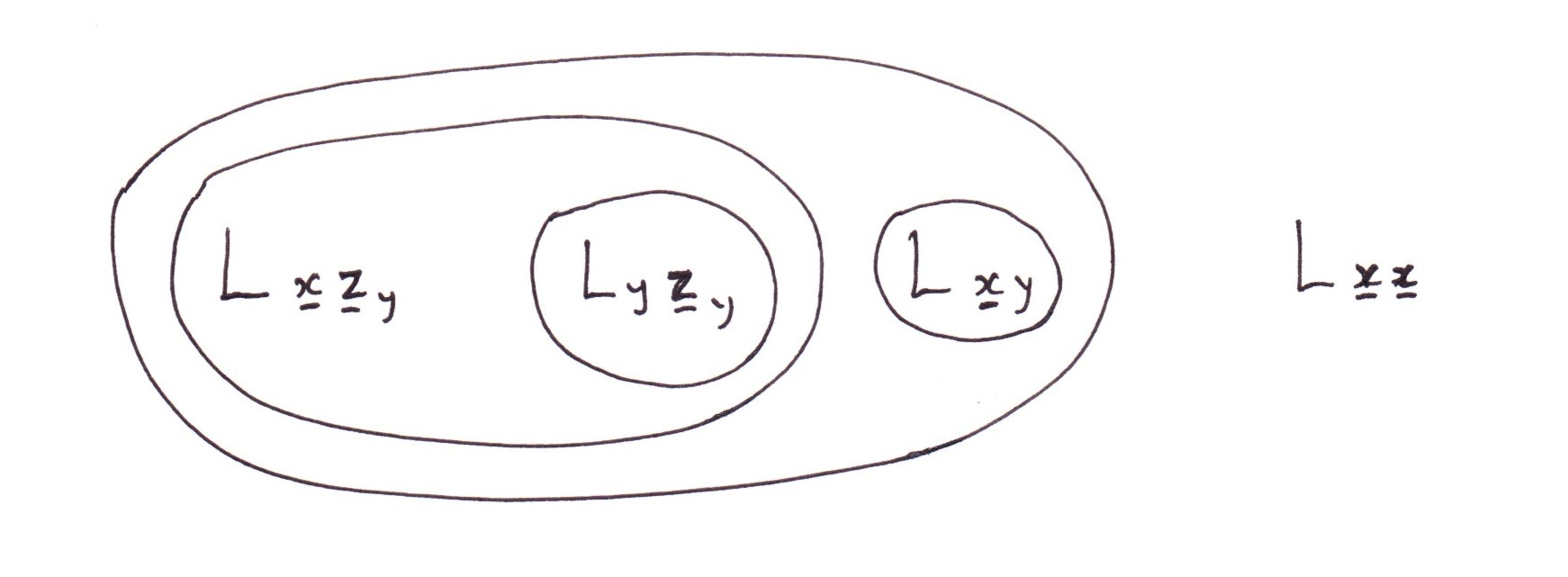Now the second factor of the expression, namely, the atom Lxx, is a transcription of the statement

x likes him or herself.

Thus the premise by itself is equivalent - algebraically - to the premise and x likes x.

### Stage 5 Appeal to a General Theorem

We're nearly there, but we haven't yet got a proof that the conclusion follows logically from the premise. For this we need to appeal to a general theorem that says that if two expressions are algebraically equivalent (as defined by the CLS rules of equivalence) then they are necessarily logically equivalent - meaning that one is true, in any particular set of circumstances, if and only if the other is true.

Such a theorem exists (for this see the main text), but requires quite a lot of machinery to prove it. For present purposes, we will take it as given.

Now, if the premise is logically equivalent to the premise and x likes x, then there can be no circumstances in which the premise is true but 'x likes x' is false. In other words, the statement 'x likes x' is implicated in - or deducible from - the premise.

Finally, given that x likes x, the sought-for conclusion ('there exists an a, such that a likes a') follows.

Now, you may well feel that all the above is a prime example of using a sledge-hammer to crack a nut. To which my reply is (i) you are quite right: general methods are indeed sledgehammers; however (ii) once you have got the sledgehammer up and running, you can use it to crack any nut. With a little practice stages 1, 2 and 3 become automatic and the work of moments. Stage 4 can be trickier, but this is where the real magic is: the reduction of 'reasoning' to 'calculation'. There are quite a few other well-established systems for accomplishing this. My claim for the Laws of Form approach is simply that it is the most economical in symbols, and therefore the most elegant, and ultimately the most beautiful.

If you want to know more, read on...

### Notes

 For completeness, although we don't need it here, we should add that the zero-place predication 'YES!!!' which is always true in all circumstances, is transcribed as the empty expression:

Thus the silence of the infinite spaces, rightly heard, connotes a resounding 'YES!!!'.

As a corollary, we note that the opposite predication 'no' (unconditional) must be transcribed as an empty circle:

O

Nyet!

Those familiar with predicate logic will recognize this as the process of 'Skolemization'.

Factor: if an expression can be seen as arising out of the juxtaposition of sub-expressions E,F,G... , in other words, can be seen as their 'product', then any of E,F,G... is a 'factor' of the whole expression.